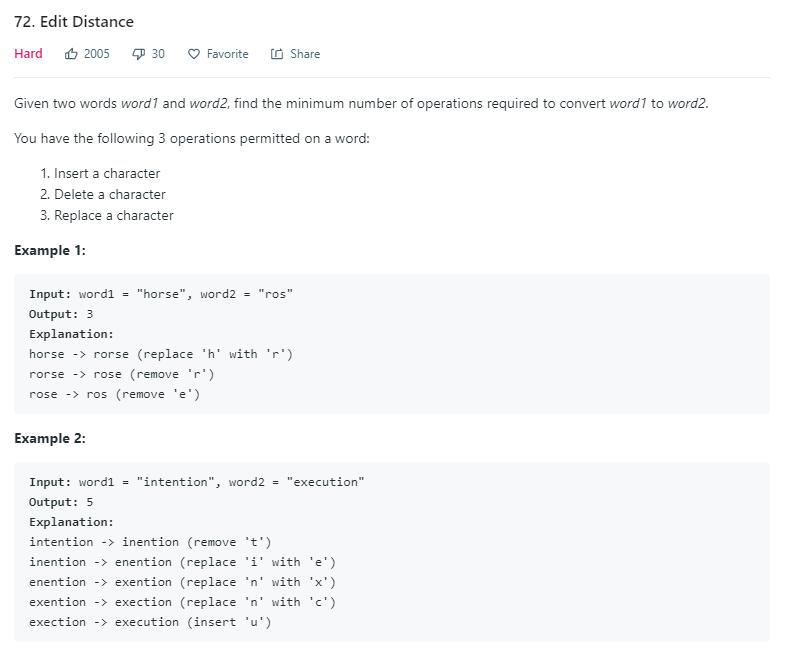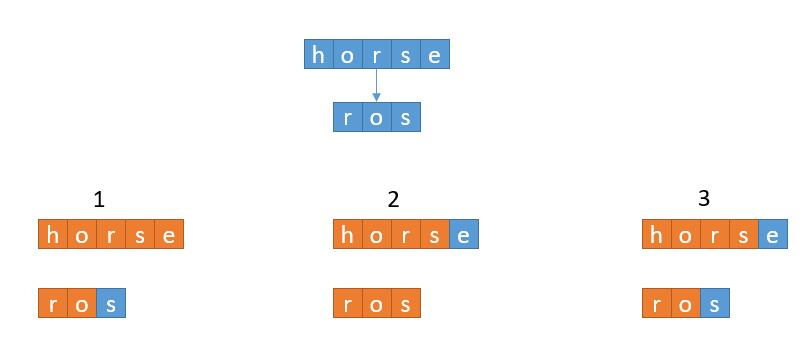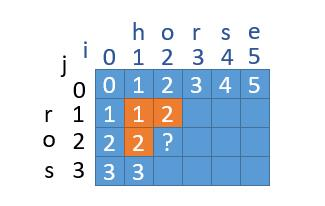# 题目描述（困难难度）# 解法一 递归public int minDistance(String word1, String word2) {
if (word1.length() == 0 && word2.length() == 0) {
return 0;
}
if (word1.length() == 0) {
return word2.length();
}
if (word2.length() == 0) {
return word1.length();
}
int x = minDistance(word1, word2.substring(0, word2.length() - 1)) + 1;
int y = minDistance(word1.substring(0, word1.length() - 1), word2) + 1;
int z = minDistance(word1.substring(0, word1.length() - 1), word2.substring(0, word2.length() - 1));
if(word1.charAt(word1.length()-1)!=word2.charAt(word2.length()-1)){
z++;
}
return Math.min(Math.min(x, y), z);
}


# 解法二 动态规划

ans[i][j] 来表示字符串 word1[ 0, i ) （word1 的第 0 到 第 i - 1个字符）和 word2[ 0, j - 1) 的最小编辑距离。然后状态转移方程就出来了。

if ( word1[m] == word2[n] )

​ ans[m][n] = Math.min ( ans[m][n-1] + 1, ans[m-1][n] + 1, ans[m-1][n-1]）

if ( word1[m] != word2[n] )

​ ans[m][n] = Math.min ( ans[m][n-1] + 1, ans[m-1][n] + 1, ans[m-1][n-1] + 1）

public int minDistance(String word1, String word2) {
if (word1.length() == 0 && word2.length() == 0) {
return 0;
}
if (word1.length() == 0) {
return word2.length();
}
if (word2.length() == 0) {
return word1.length();
}
int[][] ans = new int[word1.length() + 1][word2.length() + 1];

//把有空串的情况更新了
for (int i = 0; i <= word1.length(); i++) {
ans[i] = i;
}
for (int i = 0; i <= word2.length(); i++) {
ans[i] = i;
}
int n1 = word1.length();
int n2 = word2.length();
//从 1 开始遍历，从 0 开始的话，按照下边的算法取了 i - 1 会越界
for (int i = 1; i <= n1; i++) {
for (int j = 1; j <= n2; j++) {
int min_delete = Math.min(ans[i - 1][j], ans[i][j - 1]) + 1;
int replace = ans[i - 1][j - 1];
if (word1.charAt(i - 1) != word2.charAt(j - 1)) {
replace++;
}
ans[i][j] = Math.min(min_delete, replace);
}
}
return ans[n1][n2];
}


public int minDistance(String word1, String word2) {
if (word1.length() == 0 && word2.length() == 0) {
return 0;
}
if (word1.length() == 0) {
return word2.length();
}
if (word2.length() == 0) {
return word1.length();
}
int[][] ans = new int[word2.length() + 1];

for (int i = 0; i <= word2.length(); i++) {
ans[i] = i;
}
int n1 = word1.length();
int n2 = word2.length();
for (int i = 1; i <= n1; i++) {
//由于只用了两个数组，所以不能向以前一样一次性初始化空串，在这里提前更新 j = 0 的情况
ans[i % 2] = ans[(i - 1) % 2] + 1;
for (int j = 1; j <= n2; j++) {
int min_delete = Math.min(ans[(i - 1) % 2][j], ans[i % 2][j - 1]) + 1;
int replace = ans[(i - 1) % 2][j - 1];
if (word1.charAt(i - 1) != word2.charAt(j - 1)) {
replace++;
}
ans[i % 2][j] = Math.min(min_delete, replace);
}
}
return ans[n1 % 2][n2];
}public int minDistance(String word1, String word2) {
if (word1.length() == 0 && word2.length() == 0) {
return 0;
}
if (word1.length() == 0) {
return word2.length();
}
if (word2.length() == 0) {
return word1.length();
}
int[] ans = new int[word2.length() + 1];

for (int i = 0; i <= word2.length(); i++) {
ans[i] = i;
}
int n1 = word1.length();
int n2 = word2.length();
for (int i = 1; i <= n1; i++) {
int temp = ans;
ans = ans + 1;
for (int j = 1; j <= n2; j++) {
int min_delete = Math.min(ans[j], ans[j - 1]) + 1;
//上一列的上一个位置，直接用 temp
int replace = temp;
if (word1.charAt(i - 1) != word2.charAt(j - 1)) {
replace++;
}
//保存当前列的信息
temp = ans[j];
//再进行更新
ans[j] = Math.min(min_delete, replace);
}
}
return ans[n2];
}